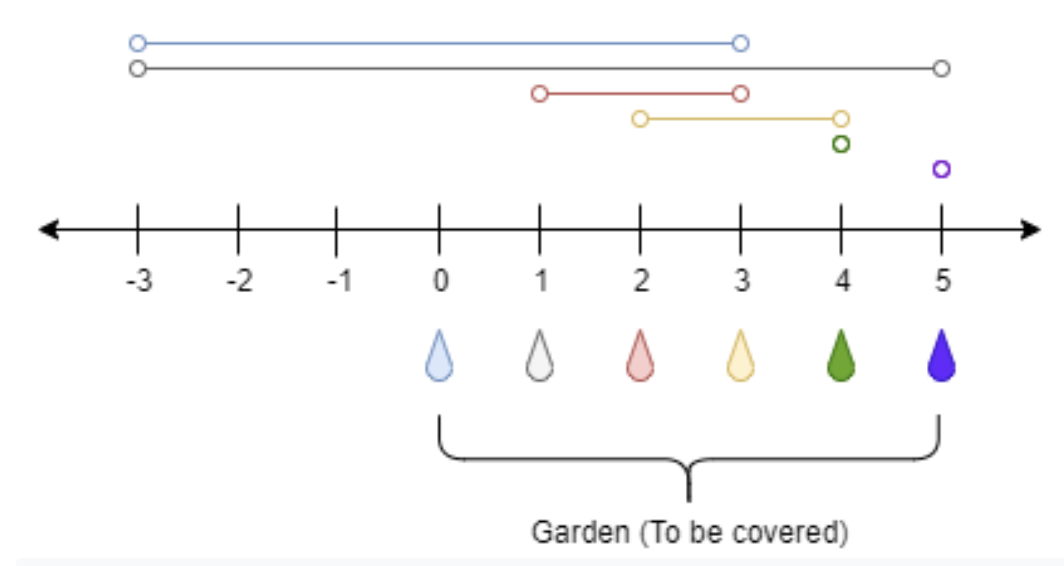# LeetCode 1326. Minimum Number of Taps to Open to Water a Garden 动态规划 离散化 贪心

There is a one-dimensional garden on the x-axis. The garden starts at the point 0and ends at the point n. (i.e The length of the garden is n).

There are n + 1 taps located at points [0, 1, ..., n] in the garden.

Given an integer n and an integer array ranges of length n + 1 where ranges[i] (0-indexed) means the i-th tap can water the area [i - ranges[i], i + ranges[i]] if it was open.

Return the minimum number of taps that should be open to water the whole garden, If the garden cannot be watered return -1.

Example 1:Input: n = 5, ranges = [3,4,1,1,0,0]
Output: 1
Explanation: The tap at point 0 can cover the interval [-3,3]
The tap at point 1 can cover the interval [-3,5]
The tap at point 2 can cover the interval [1,3]
The tap at point 3 can cover the interval [2,4]
The tap at point 4 can cover the interval [4,4]
The tap at point 5 can cover the interval [5,5]
Opening Only the second tap will water the whole garden [0,5]


Example 2:

Input: n = 3, ranges = [0,0,0,0]
Output: -1
Explanation: Even if you activate all the four taps you cannot water the whole garden.


Example 3:

Input: n = 7, ranges = [1,2,1,0,2,1,0,1]
Output: 3


Example 4:

Input: n = 8, ranges = [4,0,0,0,0,0,0,0,4]
Output: 2


Example 5:

Input: n = 8, ranges = [4,0,0,0,4,0,0,0,4]
Output: 1


Constraints:

• 1 <= n <= 10^4
• ranges.length == n + 1
• 0 <= ranges[i] <= 100

-----------------------------

思路一：动态规划

思路一比较容易想到，用f[pos]表示覆盖[0,pos]闭区间至少需要多少个水龙头，那么f[pos]=min(f[pos],f[start]+1),pos是i位置水龙头覆盖的范围[start,end]，那么代码如下：

class Solution:
def minTaps(self, n: int, ranges: List[int]) -> int:
f =  + [n+1]*n
for i in range(n+1):
start,end = max(i-ranges[i],0),min(i+ranges[i],n)
for pos in range(start, end+1):
f[pos] = min(f[pos],f[start]+1)
#print(f)
return -1 if f[n] == n+1 else f[n]


from typing import List
class Solution:
def minTaps(self, n: int, ranges: List[int]) -> int:
right_edge= *(n+1)   #key:left edge; value: right edge

for i in range(n+1):
l,r = max(0,i-ranges[i]),min(n,i+ranges[i])
right_edge[l] = r
cur_stage_end,furthest,cnt = 0,0,0
for i in range(n): #bug1: n instead of n+1
if (right_edge[i] > furthest):
furthest = right_edge[i]
if (i == cur_stage_end):
if (furthest <= cur_stage_end):
return -1
else:
cur_stage_end,furthest,cnt = furthest,i,cnt+1
return cnt

s = Solution()
print(s.minTaps(n = 7, ranges = [1,2,1,0,2,1,0,1]))

11-093794
12-1835万+02-202290
02-042201
06-011843
07-301万+
03-302576
11-072170
07-207872
09-254081